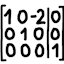#Gauss–Jordan elimination over any field

While it’s typical to solve a system of linear equations in real numbers, it’s also possible to solve a linear system over any mathematical field. Here is Java and Python code that defines various fields and provides a version of Gauss–Jordan elimination that works on any field. The basic Gauss–Jordan elimination algorithm can be adapted to solve systems of linear equations, invert matrices, calculate determinants, calculate ranks, and more.

## Source code### Java version

Core classes:

• Field.java: Defines the constants and operations that every field must support.

• Matrix.java: Represents a matrix and implements a collection of operations:

• Basic information: Dimensions, get/set cell, clone, transpose

• Row operations: Swap rows, multiply row, add two rows, multiply two matrices

• Advanced operations: Gauss–Jordan elimination, determinant, inverse

Runnable classes:

• DemoMain.java: A short demonstration program, which implements the “Rational numbers” example below.

• MatrixTest.java: A JUnit test suite for Matrix.reducedRowEchelonForm(), Matrix.determinantAndRef(), and Matrix.invert() – mostly using PrimeField(11).

Included fields:

### Python version

• fieldmath.py: The library, including the classes Field, RationalField, PrimeField, BinaryField, QuadraticSurdField, QuadraticSurd, Matrix.

• demo-main.py: A short demonstration program, which implements the “Rational numbers” example below.

• matrix-test.py: A runnable test suite for fieldmath.Matrix.reduced_row_echelon_form(), fieldmath.Matrix.determinant_and_ref(), and fieldmath.Matrix.invert() – mostly using fieldmath.PrimeField(11).

## Examples

Rational numbers

Suppose we want to solve this system of linear equations in rational numbers:

$$\begin{cases} 2x + 5y + 3z &=& 7 \\ x + z &=& 1 \\ -4x + 2y - 9z &=& 6 \end{cases}$$

First we convert the system into an augmented matrix:

$$\begin{bmatrix} 2 & 5 & 3 & 7 \\ 1 & 0 & 1 & 1 \\ -4 & 2 & -9 & 6 \end{bmatrix}$$

Then we perform Gauss–Jordan elimination on the matrix:

$$\begin{bmatrix} 1 & 0 & 0 & 67/27 \\ 0 & 1 & 0 & 35/27 \\ 0 & 0 & 1 & -40/27 \end{bmatrix}$$

And as long as the left side is the identity matrix, we can read the answer off the rightmost column:

$$\begin{cases} x &=& 67/27 \\ y &=& 35/27 \\ z &=& -40/27 \end{cases}$$

Here is the code to perform this example:

// Nice-looking matrix
int[][] input = {
{2, 5, 3, 7},
{1, 0, 1, 1},
{-4, 2, -9, 6},
};

// The actual matrix object
Matrix<Fraction> mat = new Matrix<Fraction>(
input.length, input.length, RationalField.FIELD);
for (int i = 0; i < mat.rowCount(); i++) {
for (int j = 0; j < mat.columnCount(); j++)
mat.set(i, j, new Fraction(input[i][j], 1));
}

// Gauss-Jordan elimination
mat.reducedRowEchelonForm();
Integers modulo a prime

Suppose we want to solve this system of linear equations modulo 7:

$$\begin{cases} 3x + 1y + 4z &≡& 1 \mod 7 \\ 5x + 2y + 6z &≡& 5 \mod 7 \\ 0x + 5y + 2z &≡& 1 \mod 7 \end{cases}$$

First we convert the system into an augmented matrix:

$$\begin{bmatrix} 3 & 1 & 4 & 1 \\ 5 & 2 & 6 & 5 \\ 0 & 5 & 2 & 1 \end{bmatrix}$$

Then we perform Gauss–Jordan elimination on the matrix:

$$\begin{bmatrix} 1 & 0 & 0 & 4 \\ 0 & 1 & 0 & 3 \\ 0 & 0 & 1 & 0 \end{bmatrix}$$

And as long as the left side is the identity matrix, we can read the answer off the rightmost column:

$$\begin{cases} x &≡& 4 \mod 7 \\ y &≡& 3 \mod 7 \\ z &≡& 0 \mod 7 \end{cases}$$

Here is the code to perform this example:

// Nice-looking matrix
int[][] input = {
{3, 1, 4, 1},
{5, 2, 6, 5},
{0, 5, 2, 1},
};

// The actual matrix object
Matrix<Integer> mat = new Matrix<Integer>(
input.length, input.length, new PrimeField(7));
for (int i = 0; i < mat.rowCount(); i++) {
for (int j = 0; j < mat.columnCount(); j++)
mat.set(i, j, input[i][j]);
}

// Gauss-Jordan elimination
mat.reducedRowEchelonForm();